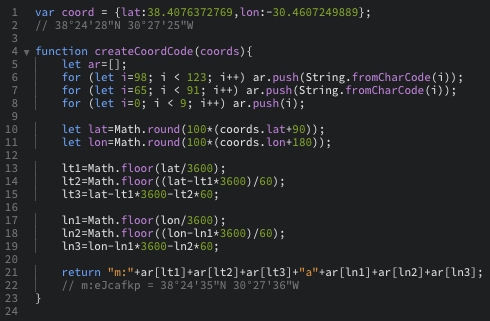# SOLVED How to create marker on URL

• This post is deleted!

• @GeGaX

JS function for you:

``````function createCoordCode(coords){
let ar=[];
for (let i=98; i < 123; i++) ar.push(String.fromCharCode(i));
for (let i=65; i < 91; i++) ar.push(String.fromCharCode(i));
for (let i=0; i < 9; i++) ar.push(i);

let lat=Math.round(100*(coords.lat+90));
let lon=Math.round(100*(coords.lon+180));

lt1=Math.floor(lat/3600);
lt2=Math.floor((lat-lt1*3600)/60);
lt3=lat-lt1*3600-lt2*60;

ln1=Math.floor(lon/3600);
ln2=Math.floor((lon-ln1*3600)/60);
ln3=lon-ln1*3600-ln2*60;

return "m:"+ar[lt1]+ar[lt2]+ar[lt3]+"a"+ar[ln1]+ar[ln2]+ar[ln3];
}

console.log(createCoordCode({lat:-29,lon:100}));
``````

• @rittels Thanks, I test it and tell you 😉

• @rittelsI have a shift of 7 second North and 11 second West between the real position and Windy position.
What should I correct in your code to obtain an identical position?
Thanks again for the code, it's cool 😉

• @GeGaX

That happens, because the max resolution of the alphanum code is 0.01deg, thus 36 seconds (about 1km).

``````function createCoordCode(coords){
let ar=[];
for (let i=98;i < 123;i++)ar.push(String.fromCharCode(i));
for (let i=65;i < 91;i++)ar.push(String.fromCharCode(i));
for (let i=0;i < 9;i++)ar.push(i);

let lat=Math.round(100*(coords.lat+90));
let lon=Math.round(100*(coords.lon+180));

return "m:"
+ar[Math.floor(lat/3600)]
+ar[Math.floor((lat%3600)/60)]
+ar[lat%60]+"a"
+ar[Math.floor(lon/3600)]
+ar[Math.floor((lon%3600)/60)]
+ar[lon%60];
}
console.log(createCoordCode({lat:38,lon:-30}));
``````

• @rittels
ok understood, thanks for everything 😉

• @rittels
In place and functional, thanks 😉

• Hi, guys we will give the option to share the picker in URL. It will be possible very soon by adding d:picker` into the URL.

• @TomSlavkovsky
Hi TomSlavkovsky,
will you share the info here when it's possible?
Thanks 😉

• @GeGaX @rittels It's now possible to open Windy with picker.

Also recommend to check following topic: https://community.windy.com/topic/77/windy-com-url-parameters

• @TomSlavkovsky
Thanks for this improvement, it works very well ;)
The GPS coordinates displayed, by the picker, are translated exactly as those sent despite the truncated display in the address bar, it's perfect !

@rittels
Thanks again for the JS code which has helped me well until here.
(And in addition I discovered the GeoHash system ;p)

• @TomSlavkovsky

Cool. Thanks!! Works well.

Can we mount plugins yet? I see we can open an airport from path.